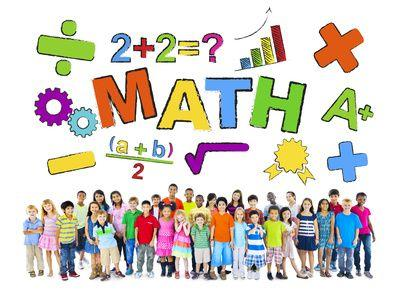# Third Grade : Free Math Worksheets

Understand the concept of place value. Learn to add, subtract, multiply, and divide bigger numbers. Go deeper into data representation, possibility, and probability of events. Move to a new level with fractions and learn about decimals. Have some fun with mixed operations, expressions, and equations.

Here is the list of all the topics that students learn in this grade. There are some sample worksheets below each section to provide a sense of what to expect. Each section has some free worksheets too. Please subscribe to access the whole content in its best form.We are aligned to common core standards. Click the button below to the see the curriculum.
Common Core

Click on any topic to view, print, or download the worksheets. All of our worksheets are free for non-commercial and personal use.

Featured Content

Number Line

Find the value of each point located on the number lines.

Category:  Place Value and Numbers     Understanding Place Value     Points on a number line

Customary Units of Length

Choose the unit you would use to measure each. Write inch, foot, yard, or mile.

Category:  Measurement     Customary Measurement     Inches, Feet, and Yards